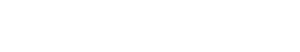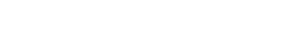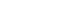# Learning Machine Learning

Machine Learning (ML; or, even more general: AI) has been available and in use for the past 30 years. Suddenly, just because of a few additions and easier-to-use libraries, various companies call it a must have? Even SAP has been participating in the hype.

The SAP Intelligent Enterprise story, Conversational AI, SAP Data Intelligence and SAP Analytics Cloud are the SAP initiatives around machine learning.

Having studied math myself, I would like to show the basics, what has changed recently, and the type of business problems machine learning can help with. (Don’t worry, all the math needed are addition and multiplication to get to the next level of understanding on ML.) At the end, it should be apparent why ML can be used for some kind of problems, has big issues with all kinds of predictions and extrapolations, and why the results are often wrong or show what was common knowledge already.

### Epilepsy

Mathematical sequences have been known for more than 2000 years. In the 70’s, they got a revival in the form of fractals. But the basic question remains the same: What happens for a given formula when the result for a provided input value is used as input value in each subsequent iteration?

Even very simple, non-linear, functions create non-obvious results. How about x² – 0.9?

If the initial value is x=2, the result of the formula is 2² – 0.9 = 4 – 0.9 =3.1. In the next iteration, this value is the new input (x=3.1). The development of this sequence is shown in the first line. After five iterations the number is very large, at iteration 11 it is larger than Excel can handle.

Starting with a value of 0.5 creates chaotic hops, as the second line shows. No pattern can be seen – although the formula, meaning the pattern, is simple. When a simple formula can create chaos, that chaos can potentially be described with simple formulas.

Our brain works like mathematical sequences as well. It can also get into a state where the numbers grow bigger and bigger until it is oversaturated. We call that an epileptic seizure.

### Intuition

To understand how the biological brain works, a simple question: There are five images in the first row, does the sixth image below match?

Intuitively, we understand the core properties of the question. However, there is some ambiguity. We could have reasoned:

• Yes, image #6 is of equal size.
• Yes, image #6 touches the border of the image at two places.
• No, image #6 is not identical to any of the five others.

Machine learning models lack such intuition entirely. As result, there is the danger that the answers are correct at the surface but do not reflect the question. In one example, a ML model was trained to recognize the dog breed. But all it learned was that huskies equal snow. Hence, the machine learning model concluded that all images with dogs in snow must mean that the dog is a husky – it learned the wrong thing.

In the above image, the question – to a human – seems to have been “Does it show a straight line from edge to edge?” But this was never explicitly explained.

Explaining the question to a ML model is done by providing examples. With a small number of examples, the probability is high that the model simply remembers what it has been offered. It is then powerful enough to retain all images in its model structure.

Therefore, either the model is made simpler (less neurons, less layers, less connections) or the number of examples is greatly increased.

Also, negative samples help to articulate the question more precisely. In our example, is touching the edge of the image a requirement, yes or no? It was never explicitly stated, but by adding images like No. 6 to the training set and categorizing them as false, the ambiguity can be removed.

### Neural Network

To solve the task of image classification, a few core properties of the network are a given: In the first layer, every pixel of the image must be connected; the output layer has two neurons showing the probability for “Yes, it is a line with our properties” and “No, it is something else”. All the layers inbetween (the propagation of the input value through the sequence) are entirely of the model designer’s own choosing.

The process to build such a network follows a pattern:

• Step 1: Scaling the values. The input image has greyscale values between 0 and 255. To avoid runaway numbers like in the Excel sheet, they get divided by 255 to rescale them to numbers between 0.00 and 1.00.
• Step 2: Input Layer. Every image is 32×32 pixel in size, hence all images can be represented by a matrix with the form (? images, 32x32x1).
• Step 3: Hidden Layers. The input is propagated through multiple layers of different types of networks. For a two-dimensional image, a Convolutional Network 2D is a good candidate. The goal is to generalize the input with as few steps as possible into two numbers for the line/no-line confidence level. In other words, the 32x32x1 numbers per image should be turned into 2 numbers. The matrix with (? images, 32x32x1) needs to be converted into a matrix of (? images, 2).
• Step 4: Pick the activation functions. Many layers by themselves are linear functions, which does not help mastering the chaos. The Conv2d layer, for example, takes a 3×3 group and adds all the nine numbers up (almost). That would be a linear behavior. For that reason, each layer gets an activation function, meaning a function like the “ReLU” (Rectified Linear Unit). That is a sophisticated name for a formula defined as “If negative then zero, else output the value as is”. A simple function, yet the overall formula is no longer linear. It also matches the biological brain: A neuron outputs an electrical signal only if the calculated output is above a threshold – the cell’s excitation potential.
• Step 5: Reduce noise. As the excitation potential is an important concept, it is added as a separate layer, the DropOut layer. This does not change the size of the matrix, but it sets all numbers below a threshold to zero, thus reducing the computational noise.

Using trial and error, the initial model is tweaked. Does the output quality degrade when simplifying the model? Which examples are not classified correctly, and therefore need more intelligence?

The ML model with a satisfying performance in quality and computer power I came up with is this:

The brain works very similarly. Via the genes, it gets an initial layout, e.g. the eye has receptors, each is connected to one neuron. These neurons add up the signals of one pixel-group to a new output, and if the output is below a threshold it gets ignored. In fact, most of the methods used in the machine learning model have a biological equivalent.

But the brain can rewire the connections, it can adapt the drop-out thresholds dynamically. Current machine learning models completely lack this plasticity of the brain.

### Settle-in

The learning efficiency is another property where biology is way ahead of any current machine learning models. Question: How often did you look at the five images to get the gist of the pattern?

In a neural network, each neuron has an initial value and the training input flows through the sequence of operations. If the output of the network is correct, the neurons supporting the decision get a boost by increasing their co-factors. The equivalent is done in case the result was incorrect. This step, the backpropagation, is repeated thousands, even millions of times until these co-factors become stable.

In this example, the accuracy of the network after each training interval is shown. The network learned the desired outcome very quickly, got better and better and the result at the end was correct for 98 percent of the input images.

### Transformations

The huge advantages neural networks provide become obvious when comparing them with other algorithms. If the question is: “Are two images identical, pixel by pixel?”, that is the domain of classic programming. But what if the image is scanned and then analyzed? What was black before is now a dark gray, it is slightly tilted, there is a speck of dust in one place.

Programming for all these variances in the data is possible but expensive. A neural network incorporates the tolerance via the way it works.

Here are a few examples of completely new images and the output of the network:

Above each image the result NOLINE/LINE is printed, and below the probability values for each. The first image is not a line and the confidence in the result is 100 percent. In the second image, it believes it is no line, either, but just by 99.1 percent.

### Predictions

The side effect of this input tolerance is, in my opinion, the reason machine learning models are believed to be more powerful than they actually are. Given what we have learned, it should be obvious by now that ML models require inputs for all situations. Yes, they can cope with input that is out of the ordinary, as opposed to classic programming. But only in the sense that a traditional program will likely return an error and quit, while a machine learning model outputs something – even if it isn’t the correct answer. In the best-case scenario, the confidence level is 50-50, and thus the indecision is visible. Machine learning often only believes to have an answer.

Looking at the last image of the test group, the line starting and ending within the image, it said that this is no line according to our definition, and its confidence level is 99.5 percent. Fine, except that the training images never had such an example. The training data included diagonal lines and ellipses. So how does it know? Where does the high level of confidence come from?

Speaking of which, what is the result for an empty image, a line along one edge, a 50 percent gray colored line or a dotted line? As none of these border-line cases (sorry for the pun) were provided during the learning phase, the output is arbitrary.

We humans have an intuitive understanding of the world around us. We understand the goal, the physics, can describe the problem on a meta level. A machine learning model can do none of these things.

This is especially interesting considering recent media coverage of autonomous cars. Will they be safer compared to a human driver? Assuming the network works flawlessly, yes. But only for situations similar to what they have seen already. If roads look similar to the training data, the weather conditions are similar, the visibility is high, the car will drive flawlessly. But: As soon as you put such a car on a snow-covered highway, for example, it will have issues.

Most importantly, how should a car react when a crash is unavoidable? What is the ethically correct decision? This question is a glorification of the artificial intelligence embedded in the car. As all it can do is learning by example and such an example was never part of the training data, it will do something, anything! Hopefully something good and not like a deer in headlights, frozen by indecision.

### Optical illusions

Coming back to the basics, if the goal is to abstract the input more and more by each layer, it could be possible that a completely different input creates the same input values at one of the lower layers.

We call this an optical illusion. Are those two red lines straight?

If humans can be misguided, then a neural network is even easier to trick.

### Bias, Cause, Effect

A huge problem is the bias of the network. As it is a mathematical sequence of operations chosen by the developer and trained with the data at hand, there is quite a high possibility to program an unintended bias into the result. Because the network is executed on a computer, it is assumed to be neutral, which is just not true even in the best of cases.

One specific type of bias is mixing up cause and effect. In a hospital, the probability of finding sick people is significantly higher than in the average population. Conclusion: Do not go into the hospital and you will be healthy. And stop getting older, too, as this increases illnesses as well.

All correct assumptions, but are they useful? Thinking further: Should we make life-and-death decisions based on such findings?

### When to use machine learning

In summary, today’s AI models are good at finding patterns; although not as good as we humans in cases where the number of dimensions is low.

Profit per region can be visualized easily. Adding the time dimension is no problem either, maybe visualized as an animated heatmap of the country, the animation showing the development over time.

But adding more and more dimensions like product type, sales channel, customer size,… would be too much. We would split the problem into smaller ones and thus miss out on cross references, e.g. that a direct sales channel is important for profit for large customers, or that the price is the main driver for individuals’ decision to buy.

Machine learning models, however, can cope with many dimensions easily.

### Types of AIs

The other point to keep in mind is that the machine learning model used in this example is one type out of many, and each has variations. Different formulas can be used to represent a neuron, e.g. a neuron with a memory of the previous values. This is required for tasks where the order of the input plays a significant role. In text data processing, for example, the phrases: “I can only do that” and “Only I can do that” do have a different meaning.

Coming back to biology, the brain is not a simple feed-forward network. The output of one neuron is connected to another and another and its output is again used as input for the first one. These kinds of loops create all sorts of issues we cannot deal with in the digital, discrete world whereas the analogue, massively parallel processing biological brain is very efficient.

We have learned a lot already and have multiple areas to explore. A specialized computer chip that is programmable but works analogue instead of digital would be an interesting option. Quantum computing might outpace even the human brain. But all of these are just optimizations. To solve a problem well, the machine learning model needs to understand the problem – and in that area, we are totally clueless.

To quote my favorite comedian Gunkl: “If knowledge is power, the most powerful people must be brilliant. Why does this conclusion collide with reality so often, then?”

##### Source:
rtdi.io#### Werner Daehn, rtdi.io

Werner Daehn, former SAP employee, is an expert on big data and smart data integration. He is also the CEO of rtdi.io.

This site uses Akismet to reduce spam. Learn how your comment data is processed.